Your data analysis solution

# RV coefficient test in Excel tutorial

2018-06-06

This tutorial will help you compute and interpret RV coefficients used to test the association between two matrices, in Excel using XLSTAT.
Not sure this is the statistical test you are looking for? Check out this guide.

## Dataset to run an RV coefficient test in XLSTAT

An Excel sheet containing both the data and the results for use in this tutorial can be downloaded by clicking here.

The data used in this example correspond to a study where 12 cranberry juices have been evaluated by two sensory panels. Each panel evaluated the product with their own lexicon. Panel FR used 6 attributes and panel CA used 7 attributes. Data were analyzed by mean of PCA.

## Goal of this tutorial

The goal of this tutorial is to evaluate the similarity between the results obtained from two PCAs.

## Setting up an RV coefficient test in XLSTAT

After opening XLSTAT, click on Test a hypothesis / Correlation/Association tests / RV coefficients.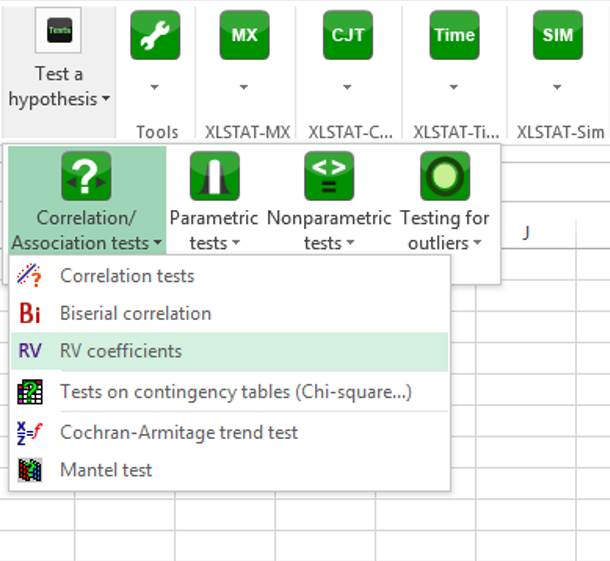In the General tab, select the first quantitative dataset (only the variables) in the Matrix A data field. Then, in the Matrix B data field, select the second dataset.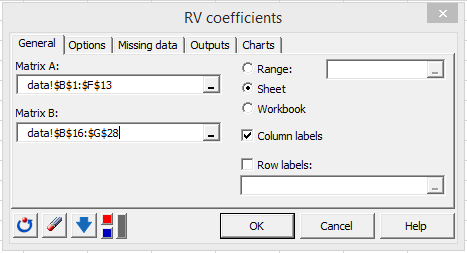In the Options tab, select the User defined option for the Selected Variables and enter 1 in the from field and 5 in the to field.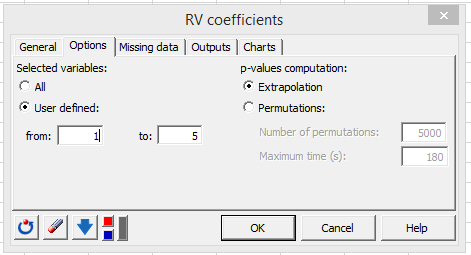Click the OK button and the Continue button in the following dialog box.

## Interpreting the results of a RV coefficient test in XLSTAT

A table displays the RV coefficients for the series of dimensions chosen, i.e. the first variable, the two first variables, the three first variables, etc. Together with the RV coefficients XLSTAT displays the adjusted RV coefficients, the standardized RV coefficients, the mean and the variance of the RV coefficient distribution and the corresponding p-values.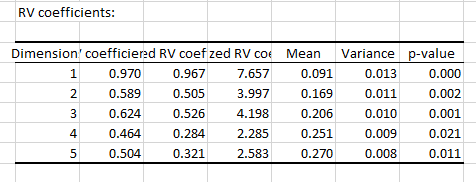The closer the RV coefficient to 1, the more similar the matrices. The lower the p-value, the more different from 0 the RV coefficient.

A bar chart allows visualizing the RV coefficients. The color code allows to quickly check if the RV coefficients are significantly different from 0 or not and at what significance level (0.001, 0.01 or 0.05).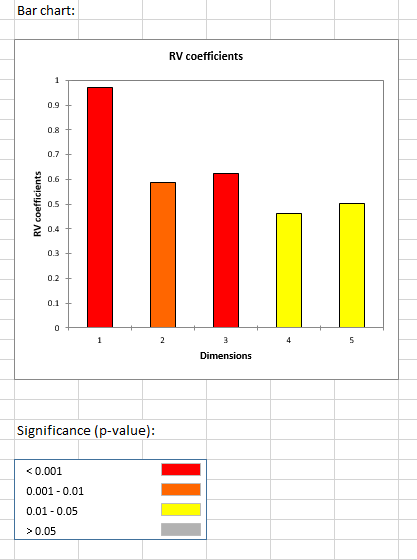### Contact Us

#### Contact our technical support team: support@xlstat.com

https://cdn.desk.com/
false
desk
Loading
seconds ago
a minute ago
minutes ago
an hour ago
hours ago
a day ago
days ago
about
false
Invalid characters found
/customer/portal/articles/autocomplete
9283